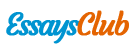# Marketing 5150

Autor:   •  April 1, 2018  •  1,257 Words (6 Pages)  •  226 Views

Page 1 of 6

...

5.

The current total contribution is \$355,000.

Contribution = Price – VC

LX1 C (\$) = 175 – 100 = 75

LX2 C (\$) = 250 – 125 = 125

LX3 C (\$) = 300 – 140 = 160

Total Contribution = (LX1 + LX2 + LX3) (Demand x Contribution)

= 2000 x 75 + 1000 x 125 + 500 x 160 = \$355,000

The profit potential for this project is \$-380 due to the costs from Model LX4, making this opportunity unfavorable.

Adjusted Demand = Prior Demand – Price x non-manufactured models

LX1 AD = 2000 – 175 x 90% = 1982

LX2 AD = 1000 – 250 x 70% = 946

LX3 AD = 500 – 300 x 40% = 392

LX4 D = 300

Total Contribution = (LX1 + LX2 + LX3 + LX4) (Demand x Contribution) - FC

= 1982 x 75 + 946 x 125 + 392 x 160 + 300 x 150 – 20,000 = \$354,620

Total profitability (\$)= 355,000 - 354,620 – 20,000 = \$-380

6. The profit generated from DC6900-Omega and

DC6900-Alpha amounts to \$685,000,000.

Unit Contribution = Price – VC

DC6900-X UC = 3900 – 1800 = 2100

DC6900-Omega UC = 5900 – 2200 = 1200

DC6900-Alpha UC = 2500 – 1200 =1300

DC6900-Omega S = 500,000 x 30% = 150,000

DC6900-Alpha S = 500,000 x 20% = 100,000

Profit (\$) = UC x Sales (models) = 3700 x 150,000 + 1300 x 100,000 = \$685,000,000

Mr Leonard should add the DC6900-X Model to the line of personal computers, as it generates additional profit of \$363,000,000 regardless of the other products.

With DC6900-X, + \$2,000,000 in FC, 500,000 sales

DC6900-X TC = 500,000 x 2100 = \$1,050,000,000

Net Profit (\$) = 555,000,000 + 130,000,000 + 1,050,000,000 – 2,000,000 = \$1,733,000,000

DC6900-X exclusive profit : 1,050,000,000 - 555,000,000 + 130,000,000 = \$363,000,000

7. a. The discounted value of the cash inflows is \$16.92 million, which is less than the present value of \$17.5 million, making this investment unfavorable.

20% Discounted value (\$) = 6.1 / 1.2 + 7.4 / 1.22 + 7/ 1.22 + 5.5 / 1.22

= \$16.92 million

b. The discounted value of the cash inflows is \$16.92 million, which is greater than the present value of \$17.5 million, making this investment favorable.

15% Discounted value (\$) = 6.1 / 1.5 + 7.4 / 1.152 + 7/ 1.153 + 5.5 / 1.154

= \$18.64 million

8. a.

The CLV is %85.36 per month.

Customer Life time value (CLV) (\$) =

Cash margin per customer (1 / (1 + interest rate – retention rate))

= (19.95 - .5 - .5) (1 / (1.01 – .788)) = \$85.36

The customer retention rate should be increased to 79%

Constant CLV with an additional \$.2 per month and variable retention rate:

\$85.36 = (\$) (19.95 - .5 - .5 -.20) (1 / (1.01 – rr))

\$4.55 = 1 / (1.01 – rr)

rr = .79 or 79%

---------------------------------------------------------------

9. a.

Pro Forma Income Statement for

Home Office System Group

Calculations

Expenses

Revenue

Sales

25,000,000

Cost of Goods Sold

7,500,000 x .4

(3,000,000)

Gross Margin

22,000,000

Marketing Expenses

Selling Expenses

25,000,000 x .15

3,750,000

(300,000 + 100,000 + (25,000,000 x .05))

1,650,000

Feright of Delivery

25,000,000 x .08

2,000,000

(7,400,000)

250,000

Depreciation and Maintenance

600,000

(300,000 + (25,000,000 x .5))

12,800,000

(13,650,000)

Net Profit

950,000

b.

Pro Forma Income Statement for

Home Office System Group